Question

# Q5. The cantilever beam, AC, is subjected to the load case shown in Figure 5. For the loading shown, do the following: [10 Marks] a) Calculate the magnitude and direction of the reactions at A b) Usi...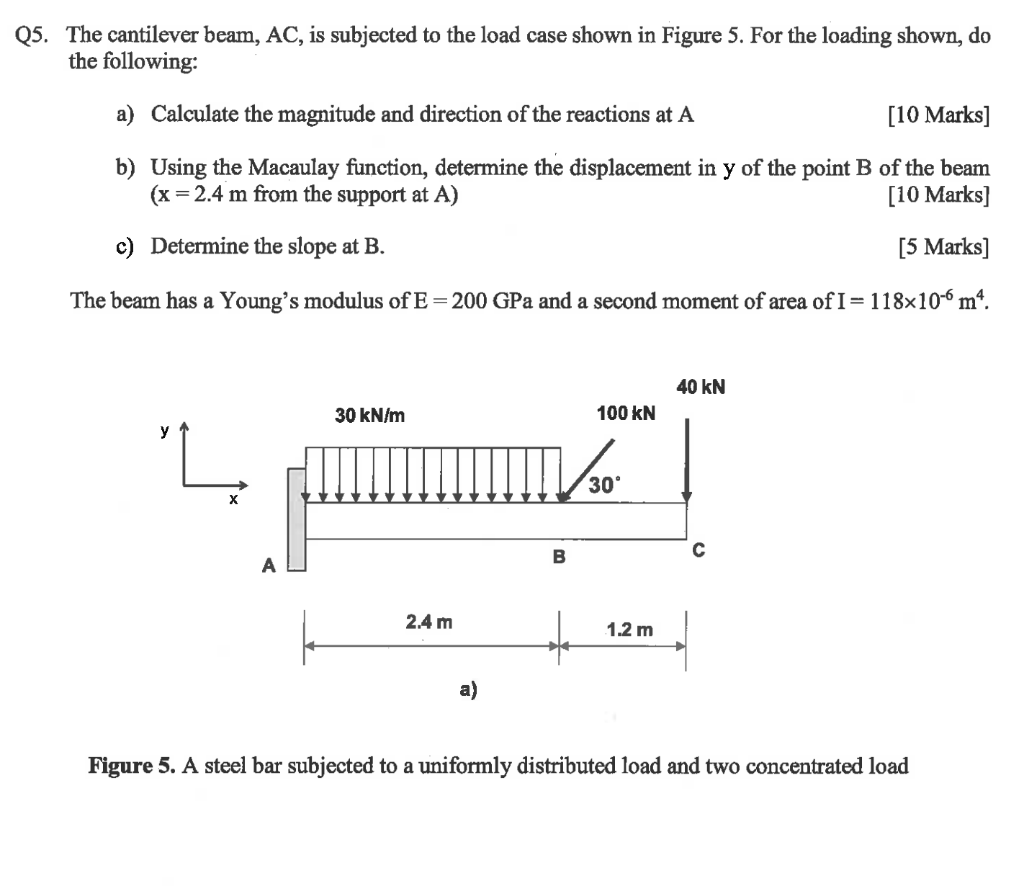Q5. The cantilever beam, AC, is subjected to the load case shown in Figure 5. For the loading shown, do the following: [10 Marks] a) Calculate the magnitude and direction of the reactions at A b) Using the Macaulay function, determine the displacement in y of the point B of the beam (x 2.4 m from the support at A) [10 Marks] c) Determine the slope at B. [5 Marks] The beam has a Young's modulus of E-200 GPa and a second moment of area of 1-1 18x10-6 m. 40 kN 100 kN 30 kN/m 30 2.4 m 1.2 m a) Figure 5. A steel bar subjected to a uniformly distributed load and two concentrated load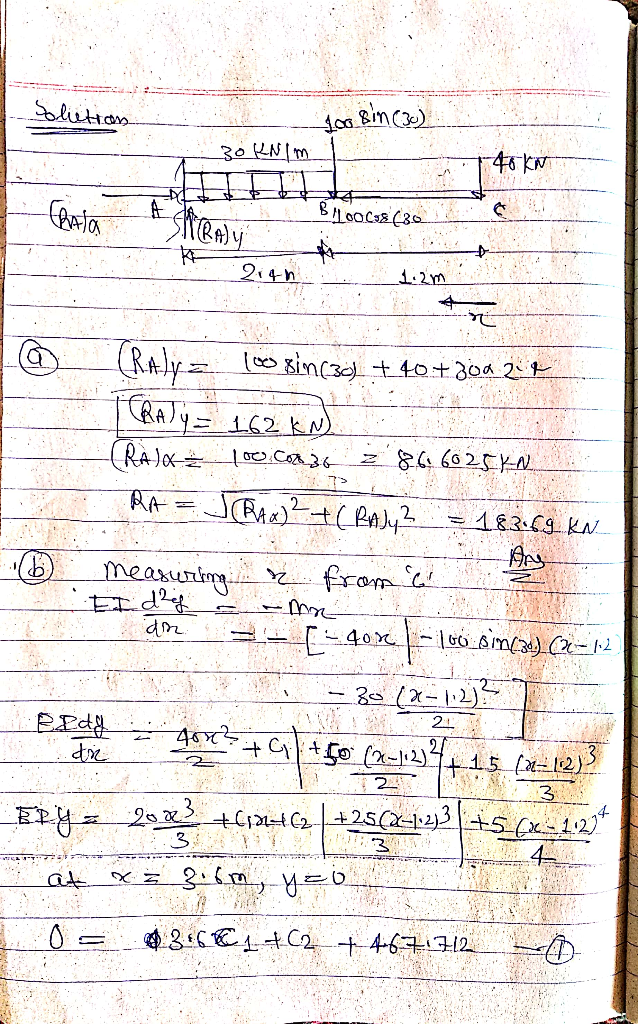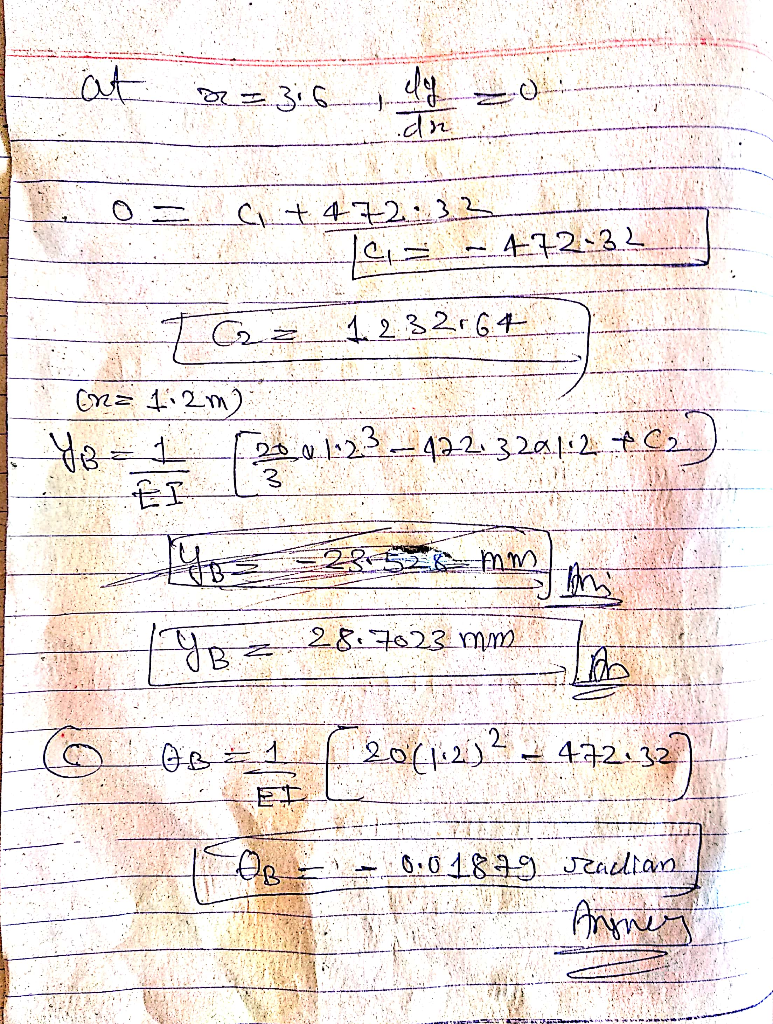#### Earn Coins

Coins can be redeemed for fabulous gifts.

Similar Homework Help Questions
• ### 8. The cantilever beam in Figure Q8 subjects to concentrated loading. The cross section geometry gives...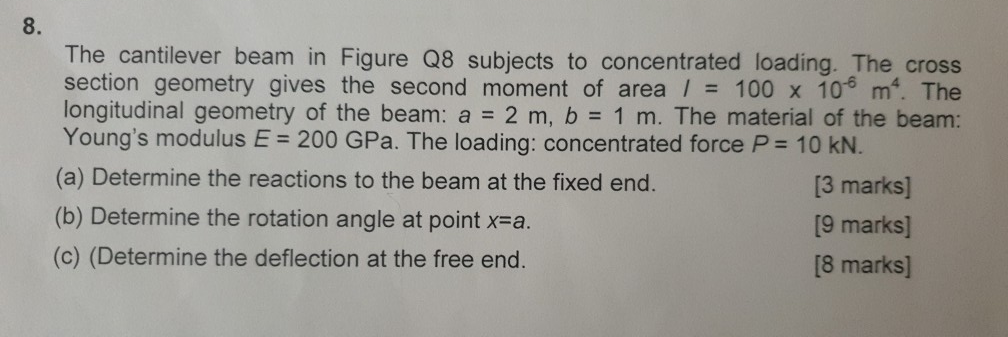8. The cantilever beam in Figure Q8 subjects to concentrated loading. The cross section geometry gives the second moment of area / 100 x 10 m. The longitudinal geometry of the beam: a 2 m, b 1 m. The material of the beam: Young's modulus E 200 GPa. The loading: concentrated force P 10 KN. (a) Determine the reactions to the beam at the fixed end. (b) Determine the rotation angle at point x-a (c) (Determine the deflection at the...

• ### A pin-connected beam AC shown in Figure is supported by 1.6m of strut BD. The beam is subjected t...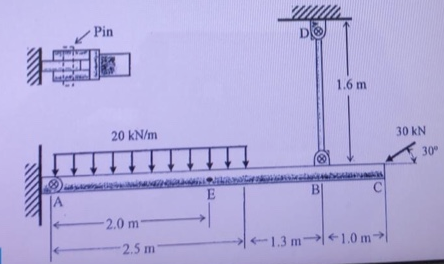A pin-connected beam AC shown in Figure is supported by 1.6m of strut BD. The beam is subjected to uniformly distributed load of 20 kN/m at 2.5m from A and an inclined concentrated load of 30 KN with 30℃ angle at respectively. The beam has a constant cross-sectional area of Abm = 0.004 m2 and the strut has a constant cross sectional area of Ast = 0.002 m2 respectively. The diameter of all pins is 20 mm. I. Determine the resultant...

• ### Q1 An elastic cantilever beam of varying cross section, as shown in Figure Q1(a), is subjected...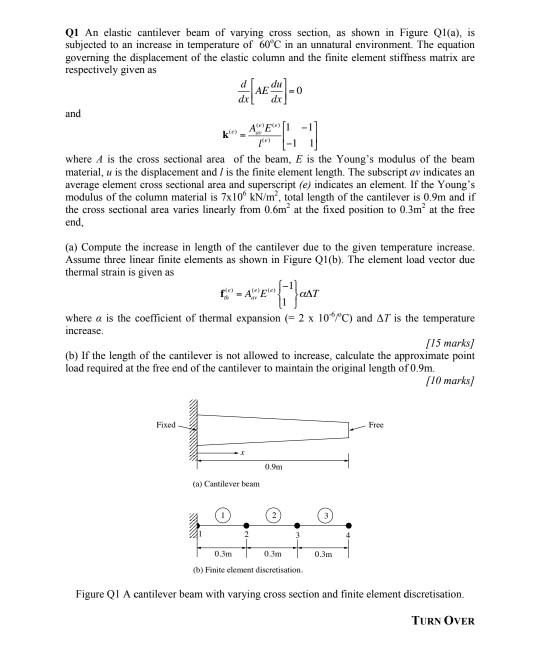Q1 An elastic cantilever beam of varying cross section, as shown in Figure Q1(a), is subjected to an increase in temperature of 60°C in an unnatural environment. The equation governing the displacement of the elastic column and the finite element stiffness matrix are respectively given as -O and ΑΕ) - where A is the cross sectional area of the beam, E is the Young's modulus of the beam material, u is the displacement and / is the finite element length....

• ### 2-The 20 foot long cantilever beam shown in the figure is subjected to the loading shown...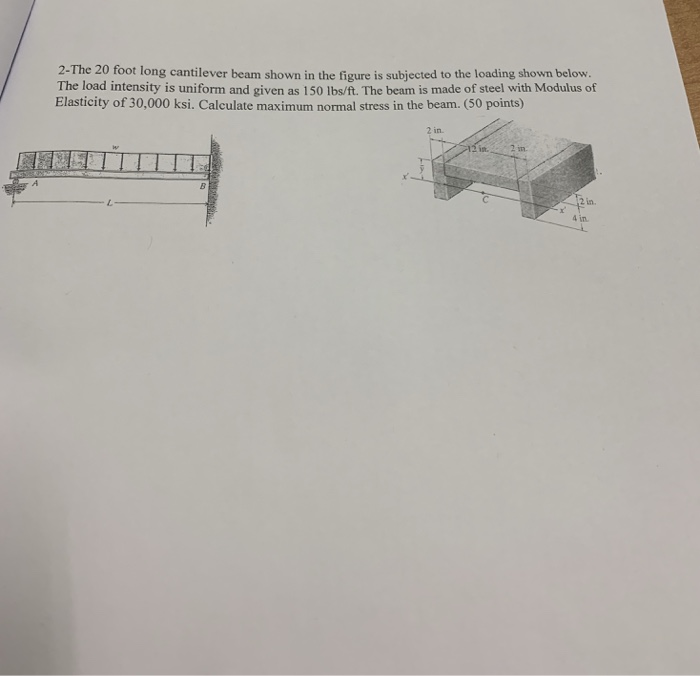2-The 20 foot long cantilever beam shown in the figure is subjected to the loading shown below. The load intensity is uniform and given as 150 lhe/ft. The beam is made of steel with Modulus of Elasticity of 30,000 ksi. Calculate maximum normal stress in the beam. (50 points) in

• ### A cantilever beam of a channel section is loaded at its half-length, as shown in Figure Q2. The Young's modulus of the material is 200 GPa. Determine the deflection at the free end. [12.5 marks]...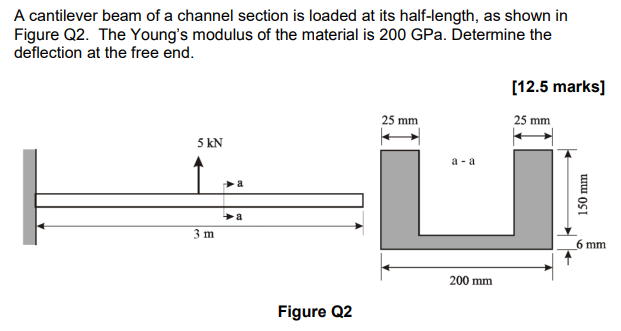A cantilever beam of a channel section is loaded at its half-length, as shown in Figure Q2. The Young's modulus of the material is 200 GPa. Determine the deflection at the free end. [12.5 marks] 25 mm 25 mm 5 kN a -a 少a 6 mm 200 mm Figure Q2 A cantilever beam of a channel section is loaded at its half-length, as shown in Figure Q2. The Young's modulus of the material is 200 GPa. Determine the deflection at...

• ### A cantilever beam AB is subjected to a triangle loading with concentrated moment (see figure). The...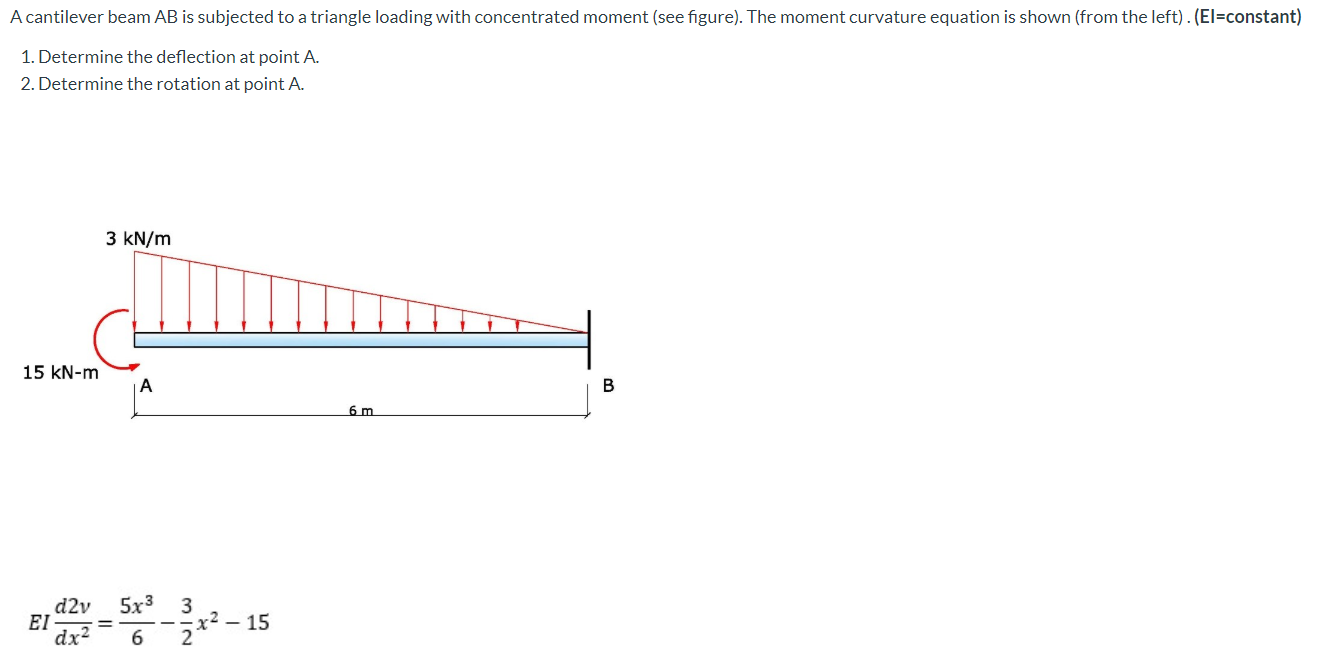A cantilever beam AB is subjected to a triangle loading with concentrated moment (see figure). The moment curvature equation is shown (from the left). (El=constant) 1. Determine the deflection at point A. 2. Determine the rotation at point A. 3 kN/m 15 kN-m A B 6 m d2v ΕΙ 5x3 3 x2 – 15 2 dx2 6

• ### The cantilever beam shown in the figure is subjected to a concentrated load at point B....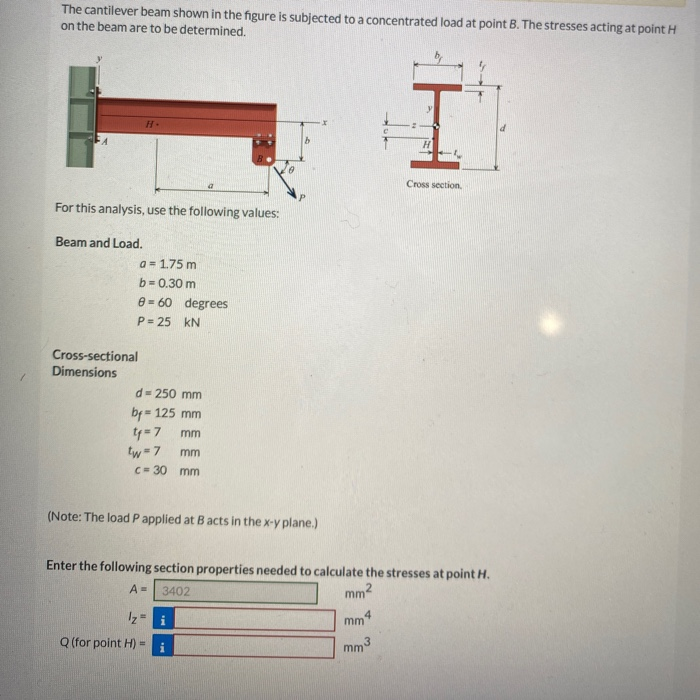The cantilever beam shown in the figure is subjected to a concentrated load at point B. The stresses acting at point H on the beam are to be determined. H Cross section For this analysis, use the following values: Beam and Load. a = 1.75 m b=0.30 m @= 60 degrees P = 25 KN Cross-sectional Dimensions d=250 mm bp = 125 mm ty=7 mm tw = 7 mm C= 30 mm (Note: The load P applied at Bacts in...

• ### For the cantilever steel beam shown below, find the deflection at point B. Q5 Assume that:...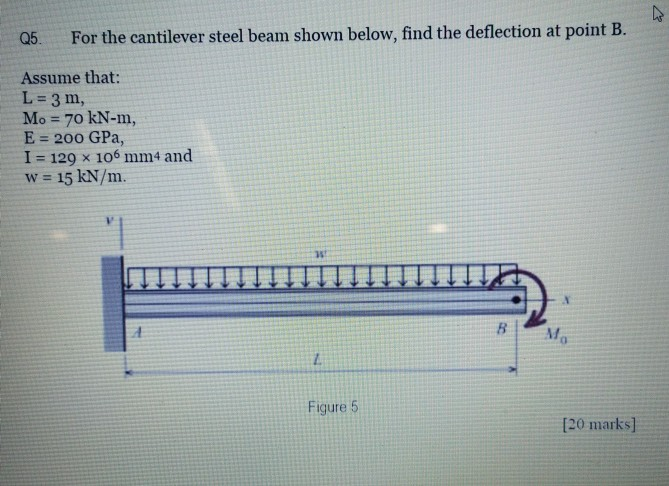For the cantilever steel beam shown below, find the deflection at point B. Q5 Assume that: L 3 m, Mo 70 kN-m, E 200 GPa, I 129 x 106 mm4 and w= 15 kN/m. Mo Figure 5 [20 marks]

• ### 1.A cantilever AD is subjected to a pure moment wa at B and uniformly distributed load of intensity w along AB and BC and as shown in Figure 1. The beam has constant EI. Ignore the weight of the bean...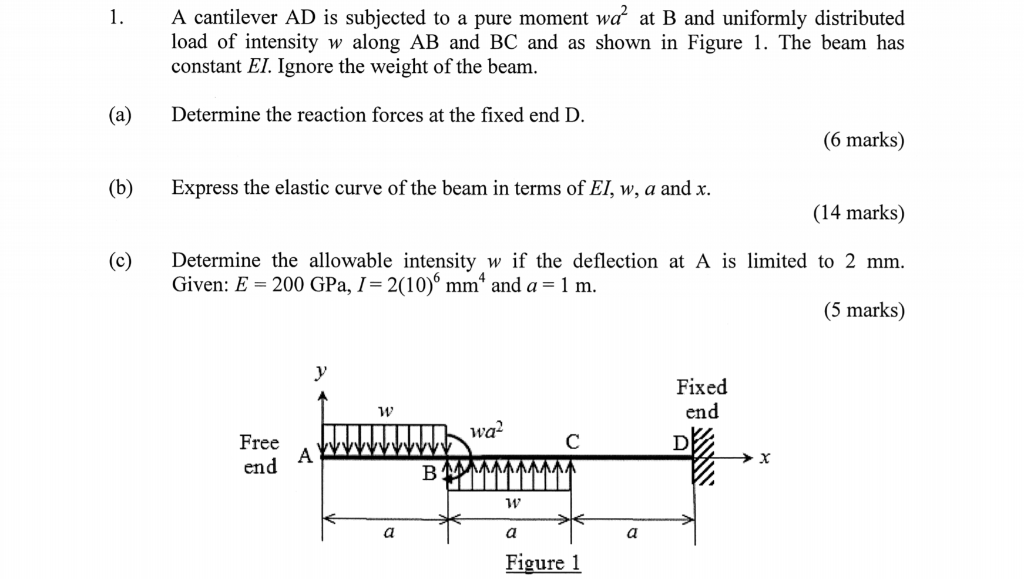1.A cantilever AD is subjected to a pure moment wa at B and uniformly distributed load of intensity w along AB and BC and as shown in Figure 1. The beam has constant EI. Ignore the weight of the beanm (a)Determine the reaction forces at the fixed end D. (6 marks) (b) Express the elastic curve of the beam in terms of EI, w, a and x. (14 marks) (c)Determine the allowable intensity w if the deflection at A is...

• ### (a) A cantilever beam shown in Figure 6 is subjected to a concentrated load P. Deflection...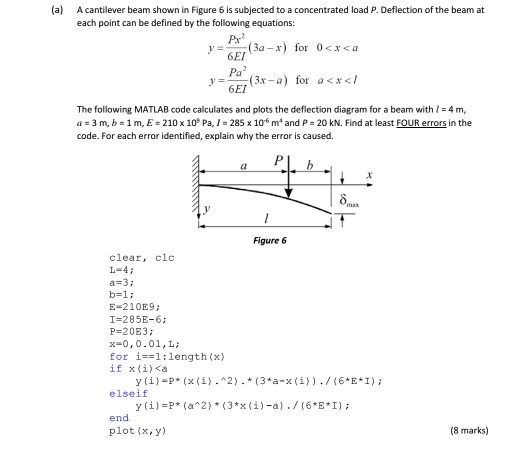(a) A cantilever beam shown in Figure 6 is subjected to a concentrated load P. Deflection of the beam at each point can be defined by the following equations: 6EI Pa 6EI F3x-a) for axx<l The following MATLAB code calculates and plots the deflection diagram for a beam with 1-4 m, d1 = 3 m, b = 1 m,E>210 x 10, Pa, 1 = 285 x 10-6 m4 and P = 20 kN. Find at least FOUR errors in the...